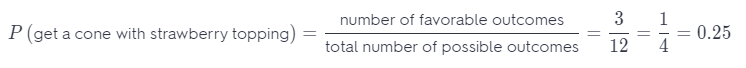Refer to our Texas Go Math Grade 7 Answer Key Pdf to score good marks in the exams. Test yourself by practicing the problems from Texas Go Math Grade 7 Module 6 Quiz Answer Key.

6.1, 6.2 Theoretical Probability of Simple and Compound Events

Find the probability of each event. Write your answer as a fraction, as a decimal, and as a percent.

Question 1.
You choose a marble at random from a bag containing 12 red, 12 blue, 15 green, 9 yellow, and 12 black marbles. The marble is red.
The number of possible outcomes is:
12 + 12 + 15 + 9 + 12 = 61
P (choose a red marble) =The probability of choosing a red marble is $$\frac{12}{61}$$.

You draw a card at random from a shuffled deck of 52 cards. The deck has four 13-card suits (diamonds, hearts, clubs, spades). The card is a diamond or a spade.
The total number of possible outcomes is: 4 ∙ 13 = 52
P (diamond or a spade) =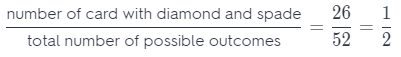The probability to draw a diamond or spide is $$\frac{1}{2}$$.

6.3 Making Predictions with Theoretical Probability

Question 3.
A bag contains 23 red marbles, 25 green marbles, and 18 blue marbles. You choose a marble at random from the bag. What color marble will you most likely choose?
The total number of possible outcomes is: 23 + 25 + 18 = 66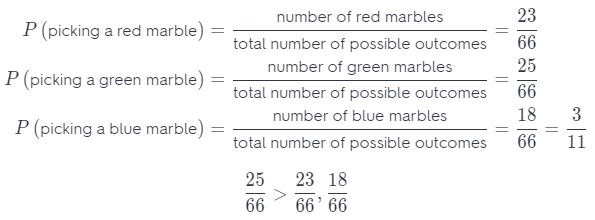It is more likely to pick a green marble than marble in other color.

6.4 Using Technology to Conduct a Simulation

Question 4.
Bay City has a 20% chance of having a flood in any given decade. The table shows the results of a simulation using random numbers to find the experimental probability that there will be a flood in Bay City in at least 1 of the next 5 decades. In the table, the number 1 represents a decade with a flood. The numbers 2 through 5 represent a decade without a flood.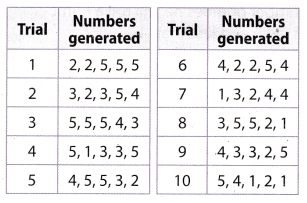According to the simulation, what is the experimental probability of a flood in Bay City in at least 1 of the next 5 decades?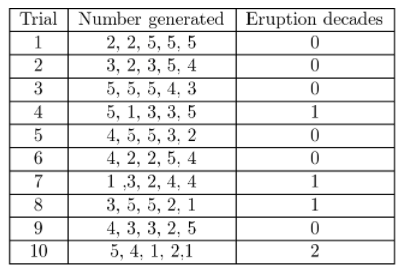The experimental probability of a flood in Bay City in at least 1 of the next 5 decades is $$\frac{4}{10}$$ = $$\frac{2}{5}$$.

Essential Question

Grade 7 Module 6 Topic Quiz Go Math Question 5.
How can you use theoretical probability to make predictions in real-world situations?
Theoretical probability is a ratio that describes what should happen, so we can make prediction about all possible situations which helps us to find the answer in real-world situations.

Texas Go Math Grade 7 Module 6 Mixed Review Texas Test Prep Answer Key

Selected Response

Question 1.
What is the probability of flipping two fair coins and having both show tails?
(A) $$\frac{1}{8}$$
(B) $$\frac{1}{4}$$
(C) $$\frac{1}{3}$$
(D) $$\frac{1}{2}$$
(B) $$\frac{1}{4}$$

Explanation:
Total numbers of possible outcomes is:
HT, HH, TT, TH = 4
Number of flips with tail on each coin The only way to fall two tails is to get tails on both coins.
P (flip on each tail) =The probability of picking two tails is $$\frac{1}{4}$$.

A bag contains 8 white marbles and 2 black marbles. You pick out a marble, record its color, and put the marble back in the bag. If you repeat this process 45 times, how many times would you expect to remove a white marble from the bag?
(A) 9
(B) 32
(C) 36
(D) 40
(A) 9 times

Explanation: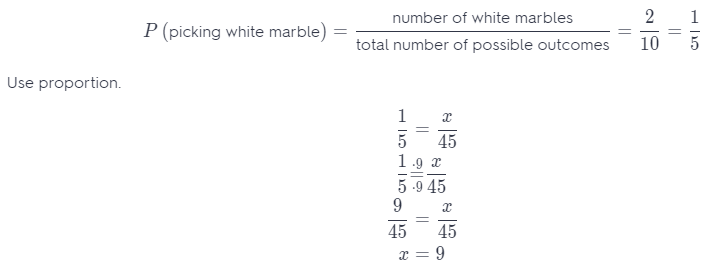You can expect to remove a white marble about 9 times.

Question 3.
Philip rolls a standard number cube 24 times. Which is the best prediction for the number of times he will roll a number that is even and less than 4?
(A) 2
(B) 3
(C) 4
(D) 6
(C) 4

Explanation:
A number that is even and less than 4 is number 2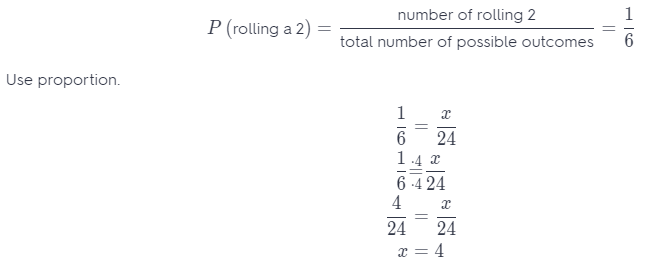The best prediction for the number of times to roll number that is even and less than 4 is 4.

Question 4.
A set of cards includes 24 yellow cards, 18 green cards, and 18 blue cards. What is the probability that a card chosen at random is not green?
(A) $$\frac{3}{10}$$
(B) $$\frac{4}{10}$$
(C) $$\frac{3}{5}$$
(D) $$\frac{7}{10}$$
(D) $$\frac{7}{10}$$

Explanation:
Total number of possible outcomes is:
24 + 18 + 18 = 60
P (choose a green card) =P (not to choose a green card) = 1 – P (choose a green card) = 1 – $$\frac{3}{10}$$ = $$\frac{10}{10}$$ – $$\frac{3}{10}$$ = $$\frac{7}{10}$$
The probability that the chosen card at random is not green is $$\frac{7}{10}$$.

A rectangle made of square tiles measures 10 tiles long and 8 tiles wide. What is the width of a similar rectangle whose length is 15 tiles?
(A) 3 tiles
(B) 12 tiles
(C) 13 tiles
(D) 18.75 tiles
(B) 12 tiles

Explanation:
Use Proportion.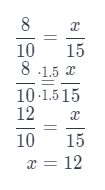The width of a similar rectangle is 12 tiles.

Question 6.
You buy a game that originally cost $35. It was on sale at 20% off. You paid 6% tax on the sale price. What was the total amount that you paid? (A)$29.68
(B) $37.10 (C)$44.10
(D) $44.52 Answer: (A)$29.68

Explanation:
Originally price is $35. Sale is 20%. Tax is 6%. Calculate how ¡iiicIi a game on sale costs. 35 – 35 ∙ $$\frac{20}{100}$$ = 35 – 35 ∙ $$\frac{1}{5}$$ = 35 – 7 =$28
Find a tax on the sale price.
28 ∙ $$\frac{6}{100}$$ = $1.68 Total amount is: sale price + tax = 28 + 1.68 =$29.68
The total amount that you paid is \$29.68.

The Fernandez family drove 273 miles in 5.25 hours. How far would they have driven at that rate in 4 hours?
(A) 208 miles
(B) 220 miles
(C) 280 miles
(D) 358 miles
(A) 208 miles

Explanation:
To find how much he drove for one hour, number of miles divide by number of hours that he drove.
$$\frac{273}{5.25}$$ = 52
He drove about 52 miles per hour
For 4 hours he will drive 4 ∙ 52 = 208 miles.
The right answer is 208 miles.

Question 8.
There are 20 tennis balls in a bag. Five are orange, 7 are white, 2 are yellow, and 6 are green. You choose one at random. Which color ball are you least likely to choose?
(A) green
(B) orange
(C) white
(D) yellow
(D) yellow

Explanation:
Total number of possible outcomes is:
5 + 7 + 2 + 6 = 20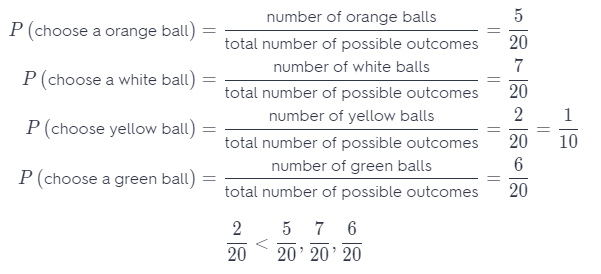It is least likely to choose a yellow ball.

Gridded Response